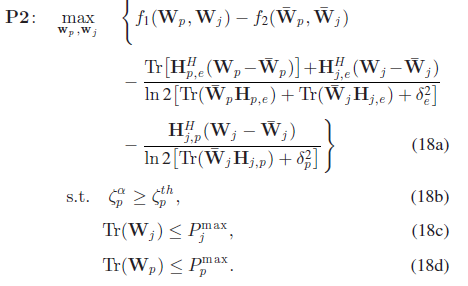# Cvx errors when coding the beamforming vector

``````clear all;
Ppmax = 30;
Pjmax=30;
Cp= 8;
Te= 0.001;
Np=-127;
B= 10;
nR=4;
nT=1;
N=1000;
for l= 1:N
h_tmp= 1/sqrt(2)*(randn(nR,nT)+1i*randn(nR,nT));
h{l}= h_tmp;
end
H{l}=h{l}*h{l}';
cvx_begin
variable w_p{nR,nR};
variable w_j{nR,nR};
w_p_bar=0;
w_j_bar=0;
W_p=w_p*w_p';
W_j=w_j*w_j';
f_1(W_p,W_j)= log2(trace(W_p*H{l})+ trace(W_j*H{l})+ Np^2)+ log2(trace(W_j*H{l})+Np^2);
f_2(W_p,W_j)= log2(trace(W_p*H{l})+ trace(W_j*H{l}+ Np^2))+ log2(trace(W_j*H{l})+Np^2);
maximize(f_1(W_p,W_j)-f_2(W_p_bar,W_j_bar)-trace(H{l}*(W_p-W_p_bar))+H{l}*(W_j-W_j_bar)/ log2(trace(W_p_bar*H{l})+trace(W_j_bar*H{l})+Np^2)-H{l}*(W_j-W_j_bar)/ log2(trace(W_j_bar*H{l})+Np^2)) ;
subject to
trace(W_p*H{l})/trace(W_j*H{l})+ Np^2 >= Cp;
trace(W_j)<= Pjmax;
trace(W_p)<=Ppmax;
cvx_end
``````

Hello everyone, I’m new to cvx and matlab. When I run this code I receive the error
Disciplined convex programming error:
Only scalar quadratic forms can be specified in CVX
.

Error in c2 (line 21)
W_p=w_p*w_p’;
w_p and w_j are beamforming vectors. Can somebody please guide me to code the beamforming vectors? Also if there are any other errors please let me know. This is my bachelor thesis and I really need the help.

is this a convex optimization problem? Have you proven it so?

Let’s look at one model piece, and use the cyclic permutation invariance of trace, which you should be familiar with, given the nature of your thesis problem, as well as the fact that `trace(X*X") = norm(X,'fro')^2`

``````log2(trace(W_p*H{l}) =
log2(trace(w_p*w_p'*h{l}*h{l}')) =
log2(trace(h{l}'*w_p*w_p'*h{l) =
log(norm(w_p'*h{l),'fro')^2)/log(2)
``````

So this reformulation avoids the “illegal” formulation of matrix times matrix transpose. But it is `log(convex)` which is illegal. So I will declare this to be a non-convex problem, unless you prove otherwise.

This reformulation would work for `trace(W_j)<= Pjmax;` (and `trace(W_j)<= Pjmax;`)

`square_pos(norm(w_p'*h{l),'fro')) <= Pjmax`
or better yet
`norm(w_p'*h{l),'fro') <= sqrt(Pjmax)`

Here is a beamforming problem in CVX. Whether this has any relation to your problem is a matter I leave to you.
https://stanford.edu/~boyd/papers/matlab/beamform_reg.m

There are some other CVX examples at http://cvxr.com/cvx/examples/ .

Here are beamforming posts on this forum http://ask.cvxr.com/search?q=beamforming . I have no idea whether there is anything relevant to you in them.

Thank you for your fast response. I will post my problem so that you can advise me how to code the maximization part accurately.the functions f1 and f2 are as follows;
f1(Wp ,Wj ) = log2(Tr(WpHp,p) + Tr(WjHj,p) + δ2
p )

• log2(Tr(WjHj,e) + δ2
e ), (12)
f2(Wp ,Wj ) = log2(Tr(WpHp,e) + Tr(WjHj,e) + δ2
e )
• log2(Tr(WjHj,p) + δ2
p ).
I also have to generate 1000 random channel vectors using monte carlo simulations and those fading coefficients used to obtain the optimum beamforming vectors wp* and wj*. How do I integrate ln2 in the objective function?
clear all;
Ppmax = 30;
Pjmax=30;
Cp= 8;
Te= 0.001;
Np=-127;
B= 10;
cvx_begin
nR=4;
nT=1;
variable W_p(nR,nR)complex;
variable W_j(nR,nR)complex;
N=1000;
for l= 1:N
h_tmp= 1/sqrt(2)(randn(nR,nT)+1irandn(nR,nT));
h{l}= h_tmp;
end
H{l}=h{l}h{l}’;
w_p_bar=0;
w_j_bar=0;
f_1(W_p,W_j)= log(trace(W_p
H{l})+ trace(W_jH{l})+ Np^2)/log(2)+ log(trace(W_jH{l})+Np^2)/log(2);
f_2(W_p,W_j)= log(trace(W_pH{l})+ trace(W_jH{l}+ Np^2))/log(2)+ log(trace(W_jH{l})+Np^2)/log(2);
maximize(f_1(W_p,W_j)-f_2(W_p_bar,W_j_bar)-trace(H{l}
(W_p-W_p_bar))+H{l}(W_j-W_j_bar)/ log(trace(W_p_barH{l})+trace(W_j_barH{l})+Np^2)/log(2)-H{l}(W_j-W_j_bar)/ log(trace(W_j_barH{l})+Np^2)/log(2)) ;
subject to
trace(W_p
H{l})/trace(W_j*H{l})+ Np^2 >= Cp;
trace(W_j)<= Pjmax;
trace(W_p)<=Ppmax;
cvx_end
my code is as above. and still receiving errors for ;
Illegal operation: log( {complex affine} )

I haven’t looked at your latest code. But I will state:

1. Is your problem convex? if not, CVX is not appropriate.

2. A cvx argument of log needs to be real. If `log(affine expression)` error message occurs, either
a) The argument would be real if conputed in exact arithmetic (i.e., without roundoff error). In such case, apply `real(...)` to the argument, so as to eliminate the roundoff level imaginary term.
or
b) The argument would be not be real if computed in exact arithmetic In such case, your model is not suitable, or in suitable form, for CVX.So my issue is, when I code this function f1 and f2 I got the error Illegal operation: log( {complex affine} ). Is there another way to code it?